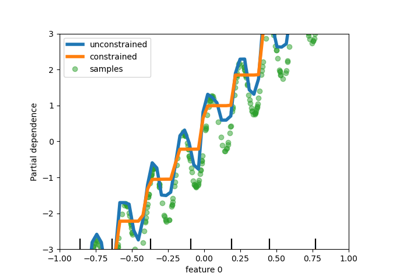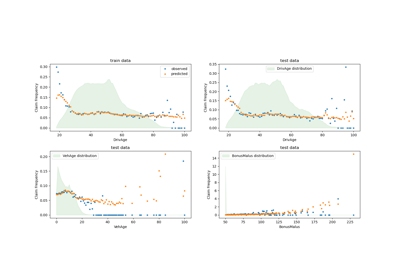# sklearn.linear_model.GammaRegressor¶

class sklearn.linear_model.GammaRegressor(*, alpha=1.0, fit_intercept=True, solver='lbfgs', max_iter=100, tol=0.0001, warm_start=False, verbose=0)[source]

Generalized Linear Model with a Gamma distribution.

This regressor uses the ‘log’ link function.

Read more in the User Guide.

New in version 0.23.

Parameters:
alphafloat, default=1

Constant that multiplies the L2 penalty term and determines the regularization strength. alpha = 0 is equivalent to unpenalized GLMs. In this case, the design matrix X must have full column rank (no collinearities). Values of alpha must be in the range [0.0, inf).

fit_interceptbool, default=True

Specifies if a constant (a.k.a. bias or intercept) should be added to the linear predictor X @ coef_ + intercept_.

solver{‘lbfgs’, ‘newton-cholesky’}, default=’lbfgs’

Algorithm to use in the optimization problem:

‘lbfgs’

Calls scipy’s L-BFGS-B optimizer.

‘newton-cholesky’

Uses Newton-Raphson steps (in arbitrary precision arithmetic equivalent to iterated reweighted least squares) with an inner Cholesky based solver. This solver is a good choice for n_samples >> n_features, especially with one-hot encoded categorical features with rare categories. Be aware that the memory usage of this solver has a quadratic dependency on n_features because it explicitly computes the Hessian matrix.

New in version 1.2.

max_iterint, default=100

The maximal number of iterations for the solver. Values must be in the range [1, inf).

tolfloat, default=1e-4

Stopping criterion. For the lbfgs solver, the iteration will stop when max{|g_j|, j = 1, ..., d} <= tol where g_j is the j-th component of the gradient (derivative) of the objective function. Values must be in the range (0.0, inf).

warm_startbool, default=False

If set to True, reuse the solution of the previous call to fit as initialization for coef_ and intercept_.

verboseint, default=0

For the lbfgs solver set verbose to any positive number for verbosity. Values must be in the range [0, inf).

Attributes:
coef_array of shape (n_features,)

Estimated coefficients for the linear predictor (X @ coef_ + intercept_) in the GLM.

intercept_float

Intercept (a.k.a. bias) added to linear predictor.

n_features_in_int

Number of features seen during fit.

New in version 0.24.

n_iter_int

Actual number of iterations used in the solver.

feature_names_in_ndarray of shape (n_features_in_,)

Names of features seen during fit. Defined only when X has feature names that are all strings.

New in version 1.0.

PoissonRegressor

Generalized Linear Model with a Poisson distribution.

TweedieRegressor

Generalized Linear Model with a Tweedie distribution.

Examples

>>> from sklearn import linear_model
>>> clf = linear_model.GammaRegressor()
>>> X = [[1, 2], [2, 3], [3, 4], [4, 3]]
>>> y = [19, 26, 33, 30]
>>> clf.fit(X, y)
GammaRegressor()
>>> clf.score(X, y)
0.773...
>>> clf.coef_
array([0.072..., 0.066...])
>>> clf.intercept_
2.896...
>>> clf.predict([[1, 0], [2, 8]])
array([19.483..., 35.795...])


Methods

 fit(X, y[, sample_weight]) Fit a Generalized Linear Model. Get metadata routing of this object. get_params([deep]) Get parameters for this estimator. Predict using GLM with feature matrix X. score(X, y[, sample_weight]) Compute D^2, the percentage of deviance explained. set_fit_request(*[, sample_weight]) Request metadata passed to the fit method. set_params(**params) Set the parameters of this estimator. set_score_request(*[, sample_weight]) Request metadata passed to the score method.
fit(X, y, sample_weight=None)[source]

Fit a Generalized Linear Model.

Parameters:
X{array-like, sparse matrix} of shape (n_samples, n_features)

Training data.

yarray-like of shape (n_samples,)

Target values.

sample_weightarray-like of shape (n_samples,), default=None

Sample weights.

Returns:
selfobject

Fitted model.

Get metadata routing of this object.

Please check User Guide on how the routing mechanism works.

Returns:

A MetadataRequest encapsulating routing information.

get_params(deep=True)[source]

Get parameters for this estimator.

Parameters:
deepbool, default=True

If True, will return the parameters for this estimator and contained subobjects that are estimators.

Returns:
paramsdict

Parameter names mapped to their values.

predict(X)[source]

Predict using GLM with feature matrix X.

Parameters:
X{array-like, sparse matrix} of shape (n_samples, n_features)

Samples.

Returns:
y_predarray of shape (n_samples,)

Returns predicted values.

score(X, y, sample_weight=None)[source]

Compute D^2, the percentage of deviance explained.

D^2 is a generalization of the coefficient of determination R^2. R^2 uses squared error and D^2 uses the deviance of this GLM, see the User Guide.

D^2 is defined as $$D^2 = 1-\frac{D(y_{true},y_{pred})}{D_{null}}$$, $$D_{null}$$ is the null deviance, i.e. the deviance of a model with intercept alone, which corresponds to $$y_{pred} = \bar{y}$$. The mean $$\bar{y}$$ is averaged by sample_weight. Best possible score is 1.0 and it can be negative (because the model can be arbitrarily worse).

Parameters:
X{array-like, sparse matrix} of shape (n_samples, n_features)

Test samples.

yarray-like of shape (n_samples,)

True values of target.

sample_weightarray-like of shape (n_samples,), default=None

Sample weights.

Returns:
scorefloat

D^2 of self.predict(X) w.r.t. y.

set_fit_request(*, sample_weight: Union[bool, None, str] = '$UNCHANGED$') [source]

Request metadata passed to the fit method.

Note that this method is only relevant if enable_metadata_routing=True (see sklearn.set_config). Please see User Guide on how the routing mechanism works.

The options for each parameter are:

• True: metadata is requested, and passed to fit if provided. The request is ignored if metadata is not provided.

• False: metadata is not requested and the meta-estimator will not pass it to fit.

• None: metadata is not requested, and the meta-estimator will raise an error if the user provides it.

• str: metadata should be passed to the meta-estimator with this given alias instead of the original name.

The default (sklearn.utils.metadata_routing.UNCHANGED) retains the existing request. This allows you to change the request for some parameters and not others.

New in version 1.3.

Note

This method is only relevant if this estimator is used as a sub-estimator of a meta-estimator, e.g. used inside a Pipeline. Otherwise it has no effect.

Parameters:
sample_weightstr, True, False, or None, default=sklearn.utils.metadata_routing.UNCHANGED

Metadata routing for sample_weight parameter in fit.

Returns:
selfobject

The updated object.

set_params(**params)[source]

Set the parameters of this estimator.

The method works on simple estimators as well as on nested objects (such as Pipeline). The latter have parameters of the form <component>__<parameter> so that it’s possible to update each component of a nested object.

Parameters:
**paramsdict

Estimator parameters.

Returns:
selfestimator instance

Estimator instance.

set_score_request(*, sample_weight: Union[bool, None, str] = '$UNCHANGED$') [source]

Request metadata passed to the score method.

Note that this method is only relevant if enable_metadata_routing=True (see sklearn.set_config). Please see User Guide on how the routing mechanism works.

The options for each parameter are:

• True: metadata is requested, and passed to score if provided. The request is ignored if metadata is not provided.

• False: metadata is not requested and the meta-estimator will not pass it to score.

• None: metadata is not requested, and the meta-estimator will raise an error if the user provides it.

• str: metadata should be passed to the meta-estimator with this given alias instead of the original name.

The default (sklearn.utils.metadata_routing.UNCHANGED) retains the existing request. This allows you to change the request for some parameters and not others.

New in version 1.3.

Note

This method is only relevant if this estimator is used as a sub-estimator of a meta-estimator, e.g. used inside a Pipeline. Otherwise it has no effect.

Parameters:
sample_weightstr, True, False, or None, default=sklearn.utils.metadata_routing.UNCHANGED

Metadata routing for sample_weight parameter in score.

Returns:
selfobject

The updated object.

## Examples using sklearn.linear_model.GammaRegressor¶Release Highlights for scikit-learn 0.23

Release Highlights for scikit-learn 0.23Tweedie regression on insurance claims

Tweedie regression on insurance claims